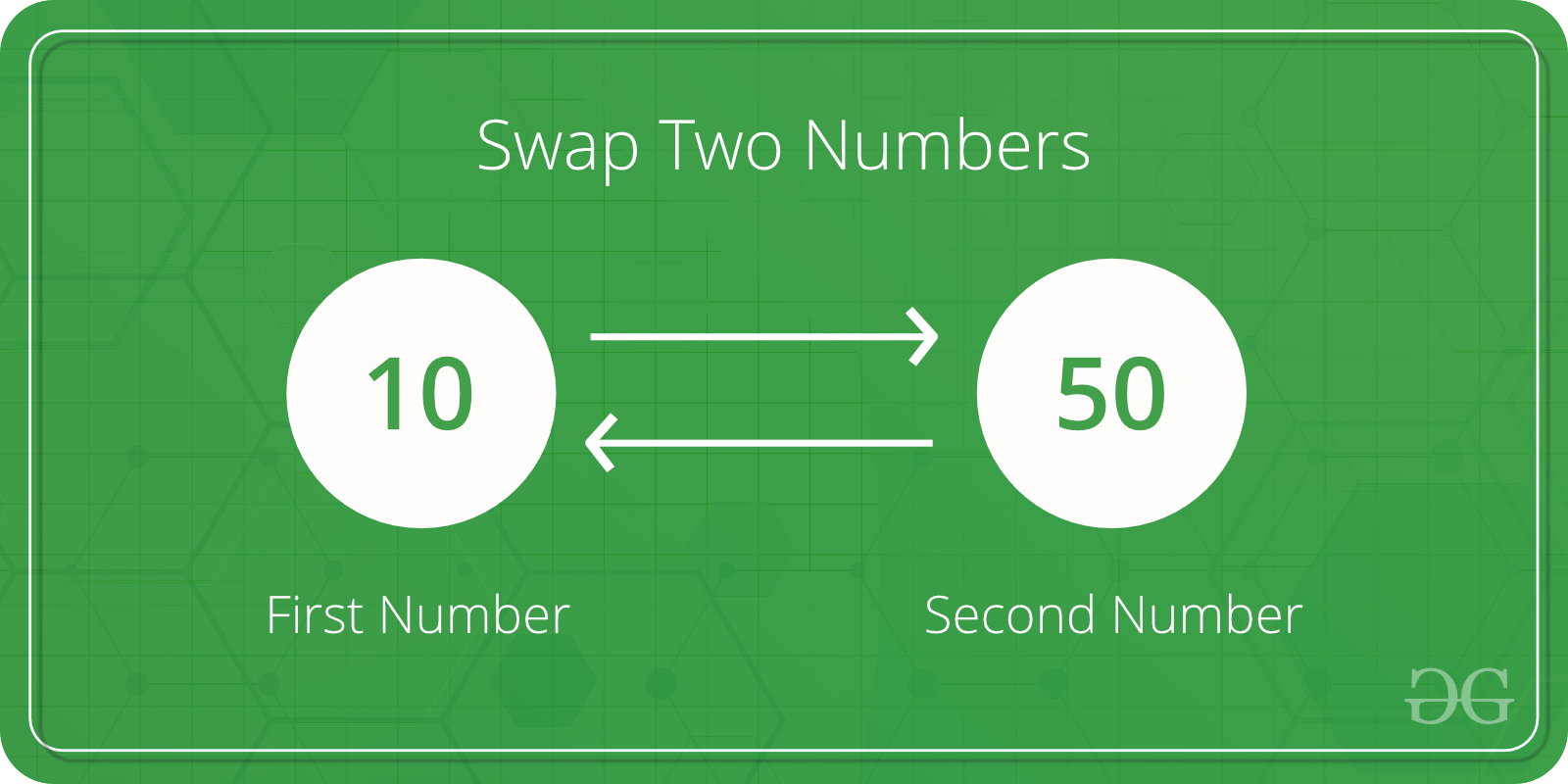# Python Program to Swap Two Variables

Given two variables x and y, write a Python program to swap their values. Let’s see different methods in Python to do this task.Method 1: Using Naive approach

The most naive approach is to store the value of one variable(say x) in a temporary variable, then assigning the variable x with the value of variable y. Finally, assign the variable y with the value of the temporary variable.

 `# Python program to demonstrate ` `# swapping of two variables ` ` `  `x ``=` `10` `y ``=` `50` ` `  `# Swapping of two variables ` `# Using third variable ` `temp ``=` `x ` `x ``=` `y ` `y ``=` `temp ` ` `  `print``(``"Value of x:"``, x) ` `print``(``"Value of y:"``, y) `

Output:

```Value of x: 50
Value of y: 10
```

Method 2: Using comma operator

Using the comma operator the value of variables can be swapped without using a third variable.

 `# Python program to demonstrate ` `# swapping of two variables ` ` `  ` `  `x ``=` `10` `y ``=` `50` ` `  `# Swapping of two variables  ` `# without using third variable ` `x, y ``=` `y, x ` ` `  `print``(``"Value of x:"``, x) ` `print``(``"Value of y:"``, y) `

Output:

```Value of x: 50
Value of y: 10
```

Method 3: Using XOR

The bitwise XOR operator can be used to swap two variables. The XOR of two numbers x and y returns a number which has all the bits as 1 wherever bits of x and y differ. For example XOR of 10 (In Binary 1010) and 5 (In Binary 0101) is 1111 and XOR of 7 (0111) and 5 (0101) is (0010).

 `# Python program to demonstrate ` `# Swapping of two variables ` ` `  `x ``=` `10` `y ``=` `50` ` `  `# Swapping using xor ` `x ``=` `x ^ y ` `y ``=` `x ^ y ` `x ``=` `x ^ y ` ` `  `print``(``"Value of x:"``, x) ` `print``(``"Value of y:"``, y) `

Output:

```Value of x: 50
Value of y: 10
```

Method 4: Using arithmetic operators

The idea is to get sum in one of the two given numbers. The numbers can then be swapped using the sum and subtraction from sum.

 `# Python program to demonstrate ` `# swapping of two variables ` ` `  `x ``=` `10` `y ``=` `50` ` `  `# Swapping of two variables ` `# using arithmetic operations ` `x ``=` `x ``+` `y    ` `y ``=` `x ``-` `y   ` `x ``=` `x ``-` `y  ` ` `  `print``(``"Value of x:"``, x) ` `print``(``"Value of y:"``, y) `

Output:

```Value of x: 50
Value of y: 10
```

My Personal Notes arrow_drop_upCheck out this Author's contributed articles.

If you like GeeksforGeeks and would like to contribute, you can also write an article using contribute.geeksforgeeks.org or mail your article to contribute@geeksforgeeks.org. See your article appearing on the GeeksforGeeks main page and help other Geeks.

Please Improve this article if you find anything incorrect by clicking on the "Improve Article" button below.

Article Tags :

Be the First to upvote.

Please write to us at contribute@geeksforgeeks.org to report any issue with the above content.